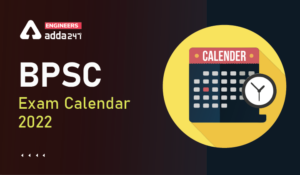Engineering Jobs   »   Civil Engineering quizs   »   UPSSSC JE QUIZ

# UPSSSC-JE’21 CE: Daily Practice Quiz. 11-October-2021

Know your strengths and practice your concepts with this quiz on UPSSSC-JE Recruitment 2021. This quiz for UPSSSC-JE Recruitment 2021 is designed specially according to UPSSSC-JE Syllabus 2021.

Quiz: Civil Engineering
Exam: UPSSSC-JE
Topic: MISCELLANEOUS

Each question carries 1 mark
Negative marking: 1/4 mark
Time: 8 Minutes

Q1. Due to rise in temperature, the viscosity and unit weight of the percolating fluid are reduced to 70% and 90% respectively. Other things being constant, the change in the coefficient of permeability will be
(a) 20%
(b) 28.6%
(c) 63%
(d) 77.8%

Q2. If a member is subjected to tensile stress of ‘P_x’ compressive stress of ‘P_y’ and tensile stress of ‘P_z^’, along the X, Y and Z directions respectively, then the resultant strain ‘E_x’ along the X direction would be (E is young’s modulus of elasticity and ‘μ’ is poison ratio)
(a) 1/E(P_x+μP_y-μP_z)
(b) 1/E (P_x+μP_y+μP_z)
(c) 1/E (P_x-μP_y+μP_z)
(d) 1/E (P_x-μP_y-μP_z)

Q3. If a shaft of diameter D is subjected to a torque T, the maximum shear stress is
(a) 64T/πD³
(b) 32T/πD³
(c) 16T/πD³
(d) 8T/πD³

Q4.The deflection of any rectangular beam simply supported is
(a) Directly proportional to its weight
(b) Inversely proportional to its weight
(c) Inversely proportional to the cube of its depth
(d) None of these

Q5. Principal planes are subjected to
(a) normal stresses only
(b) tangential stresses only
(c) normal stresses as well as tangential stresses
(d) none of these

Q6. The brick work is measured in square meter, in case of
(a) Honey comb brick work
(b) Brick flat Soling
(c) Half brick walls or the partition
(d) All options are correct

SOLUTION

S1. Ans.(b)
Sol. Before rise in temperature, K=1/C 1/s^2 yω/μ e^3/(1+e)
After rise in temperature, yω=0.9 yω
μ = 0.7 μ
Then K’ = 1/C 1/S^2 0.9yω/0.7μ e^3/(1+e)
▭(K^’=9/7 K)
Now,
Percentage change in Permeability = (9/7 K-K)/K×100
=2/7×100
=28.57≈28.6%

S2. Ans.(a)
Sol.Ex=Px/E-μ((-Py)/E)-μ(Pz/E)
▭(Ex=1/E [Px+μPy-μPz] )
E=young’s modules of elasticity
μ =Poisson’s ratio
S3. Ans.(c)
Sol. Shear stress distribution due to torque (T) for solid circular shaft of diameter (D) is-

Form torsion eq^n
T/J=τ_max/r
τ_max=T/(J\/r)
=T/z_p
T/(πD^3 \/16)
▭(τ_max=16T/(πD^3 ))

S4. Ans.(c)
Sol.Deflection (∆) = (wl^3)/48EI
∆ =(wl^3)/(48E×((bd^3)/12) ) (∵I=(bd^3)/12)
▭(∆=(wl^3)/(4Ebd^3 ))
▭(∆ α 1/d^3 )

S5. Ans.(a)
Sol. There are infinite plane passes through a single point and out of these infinites plane there are three plane which are mutually perpendicular to each other and having zero shear stress, that plane is called principal plane.

S6. Ans.(d)
Sol. Generally, the brick work is measured in terms of m³. but in case of honey comb brick work, brick flat soling, half brick wall or partition wall then, it is measured in m².

Sharing is caring!

Thank You, Your details have been submitted we will get back to you.
•Indian Air Force Apprentice Recruitment ...
•BPSC Exam Calendar 2022, Check BPSC Assi...
•SSC Scientific Assistant IMD Recruitment...
•SSC JE 2022 Apply Online, Link Activated...
•How to Clear SSC JE 2022 Electrical Engi...
•JKPSC AE Cutoff 2022, Check Here to Know...